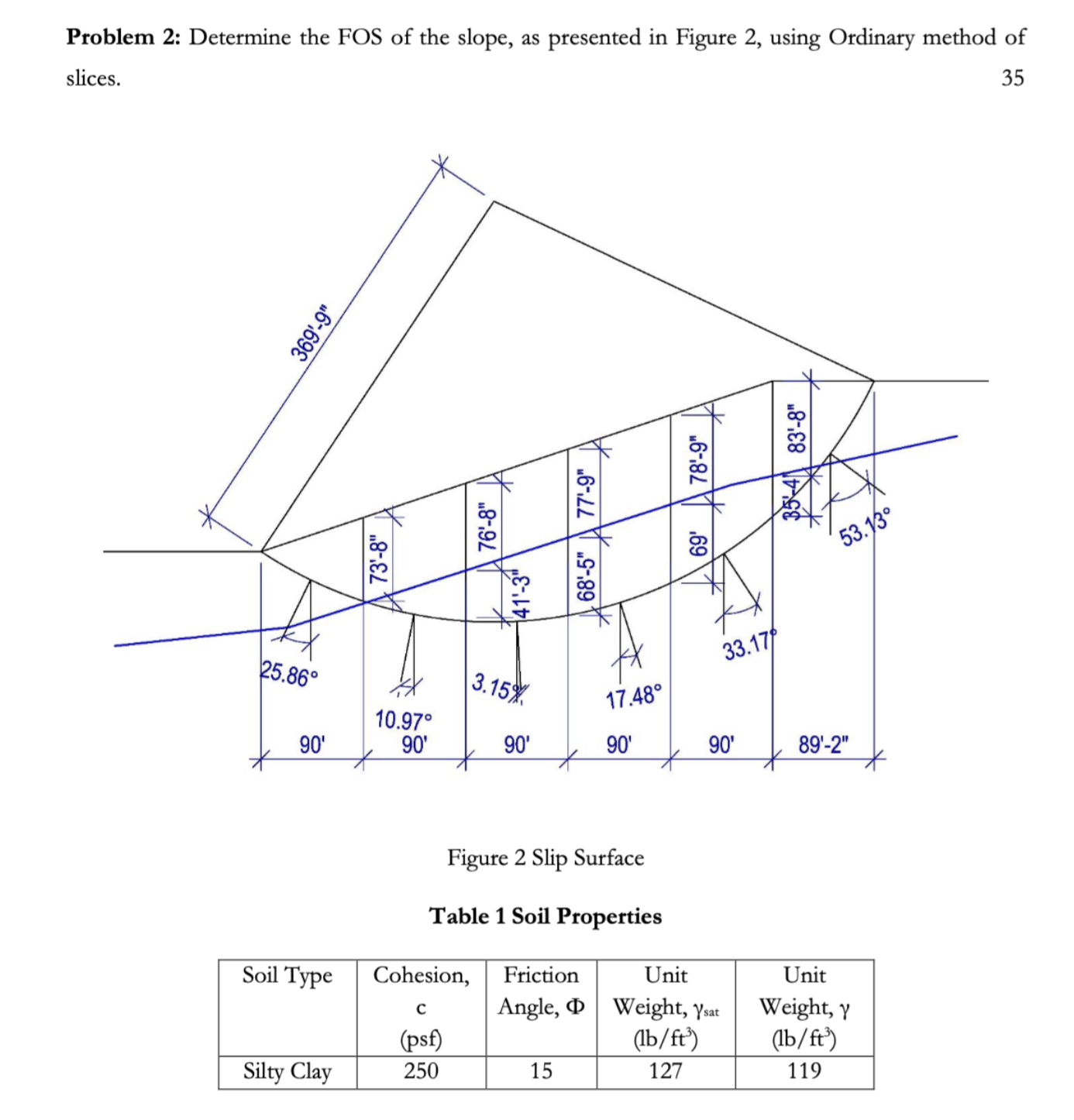Home / Expert Answers / Civil Engineering / problem-2-determine-the-fos-of-the-slope-as-presented-in-figure-2-using-ordinary-method-of-slices-pa972

# (Solved): Problem 2: Determine the FOS of the slope, as presented in Figure 2, using Ordinary method of slices ...Problem 2: Determine the FOS of the slope, as presented in Figure 2, using Ordinary method of slices. 35 Figure 2 Slip Surface Table 1 Soil Properties Soil Type Cohesion, c (psf) Friction Angle, Phi Unit Weight, gamma_("sat ") (lb//ft^(3)) Unit Weight, gamma (lb//ft^(3)) Silty Clay 250 15 127 119

Problem 2: Determine the FOS of the slope, as presented in Figure 2, using Ordinary method of slices. 35 Figure 2 Slip Surface Table 1 Soil Properties

We have an Answer from Expert